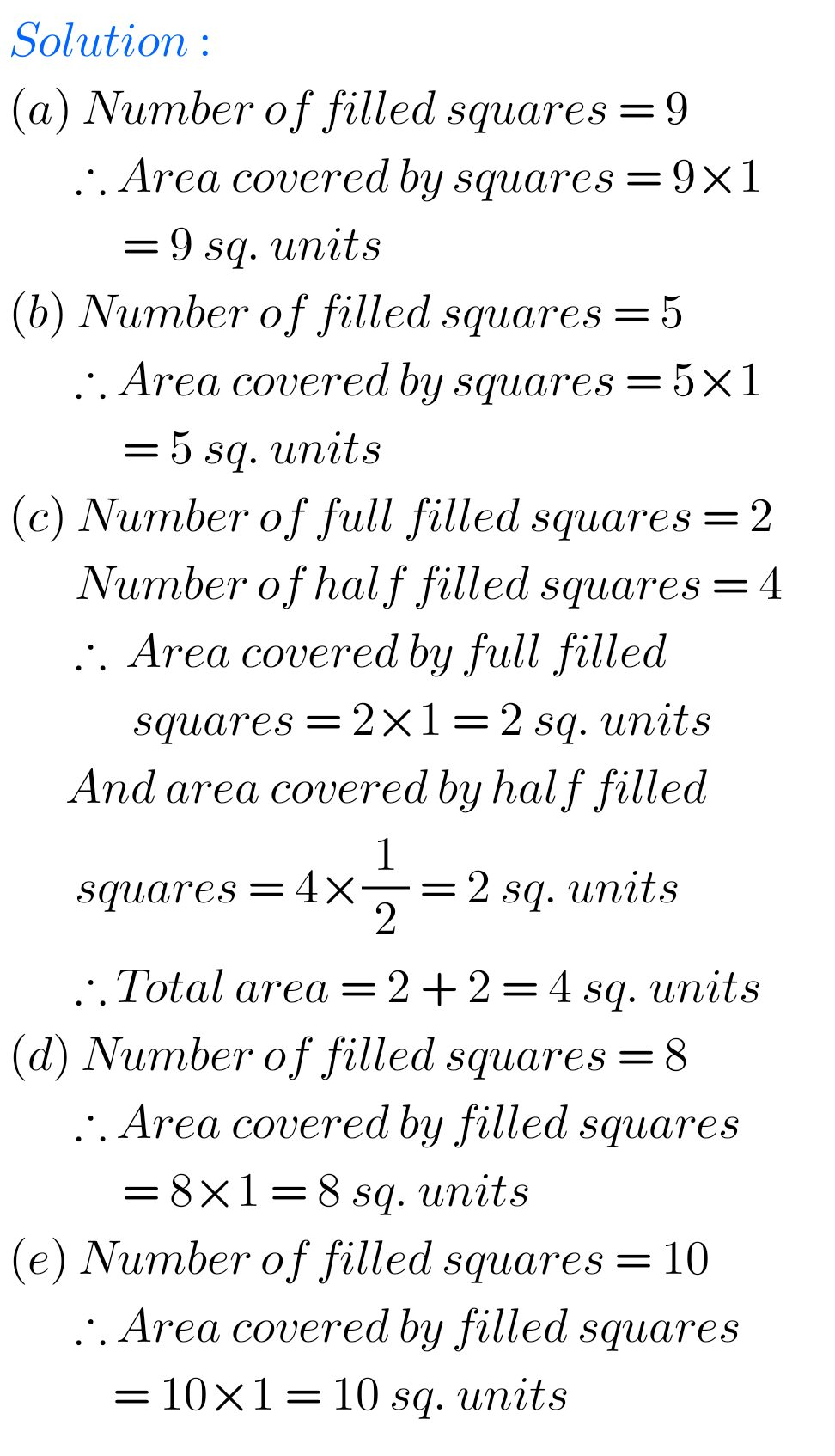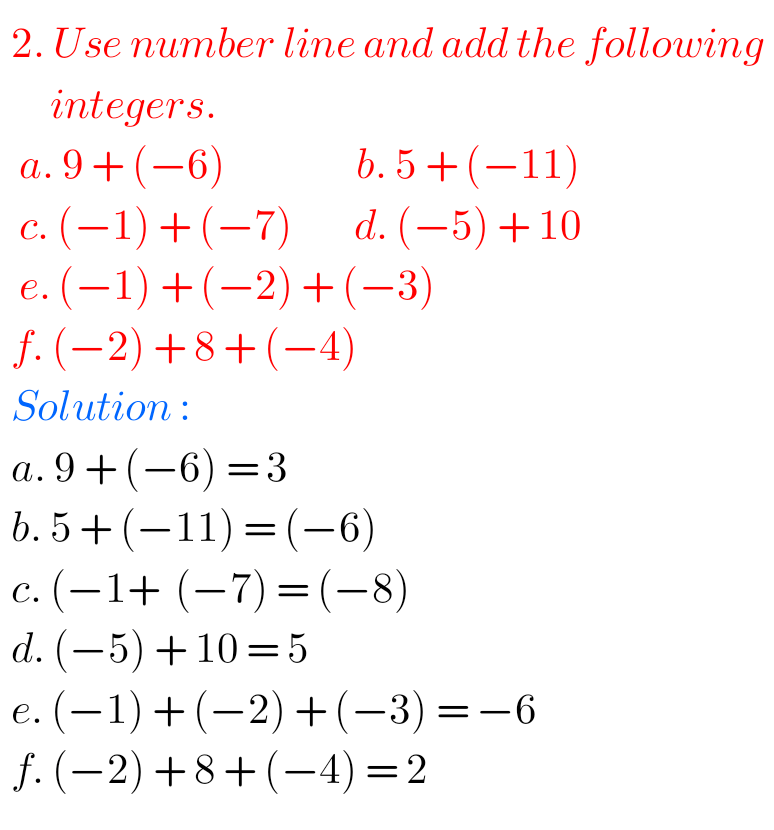# NCERT Solutions for class 6 maths## NCERT Maths solutions for Ratios and Proportions chapter 12 exercise 12.3 Class 6

Class 6 Chapter 12 exercise 12.3 solutions NCERT maths Ratios and Proportions NCERT maths class 6 chapter 12 Ratios and Proportions exercise 12.3 solutions are given. You should study the textbook lesson Ratios and Proportions very well. You should also observe, and practice example problems and solutions given in the textbook. Observe the given below …## NCERT solutions for maths class 6 chapter 12 exercise 12.2 Ratios and Proportions

Chapter 12 exercise 12.2 solutions NCERT maths class 6 Ratios and Proportions NCERT maths class 6 chapter 12 Ratios and Proportions exercise 12.2 solutions are given. You should study the textbook lesson Ratios and Proportions very well. You should also observe, and practice example problems and solutions given in the textbook. Observe the given below …

## NCERT solutions for maths class 6 exercise 12.1 Ratios and Proportions chapter 12

Ratio and Proportion Exercise 12.1 solutions NCERT Maths Class 6 NCERT class 6 Mathematics chapter 12 Exercise 12.1 Ratio and Proportion solutions are given. You should observe and practice all example problems and solutions given in the textbook. Observe the solutions and try them in your own method. NCERT solutions for maths Class 6 Ratios …## Mensuration Exercise 10.2 solutions chapter 10 class 6 ncert maths

NCERT solutions class 6 maths exercise 10.2 Mensuration Class 6 Maths NCERT Chapter 10 Mensuration Exercise 10.2 solutions are given. First you should study the textbook lesson Mensuration very well. You should also observe the example problems and solutions given in the textbook. Observe the given solutions and try them in your own method. You …

## Ncert maths solutions for class 6 chapter 9 exercise 9.1 Data Handling

Data Handling Solutions Class 6 Exercise 9.1 NCERT Maths Class 6 Maths NCERT Chapter 9 Data Handling Exercise 9.1 solutions are given. Study the textbook lesson Data Handling very well. Practice the example problems and solutions given in the textbook. Observe the given solutions and try them in your own method. NCERT class 6 maths …## NCERT Maths Solutions for Exercise 6.3 Integers class 6 Chapter 6

NCERT mathematics class 6-chapter 6 Integers Exercise 6.3 solutions are given for you. You should study chapter 6 Integers very well in the textbook. You can also observe and practice all example problems and solutions given in the textbook. Observe the solutions given below and solve them in your own way. You can also see. …## INTEGERS Exercise 6.2 solutions Chapter 2 maths class 6 NCERT

NCERT mathematics class 6-chapter 6 Integers Exercise 6.2 solutions are given. You should study chapter INTEGERS very well in the textbook. You can also observe and practice all example problems and solutions given in the textbook. Observe the solutions given below and solve them in your own way. You can also see. NCERT class 6 …

## Playing with Numbers class 6 maths exercise 3.7 solutions NCERT

NCERT mathematics class 6 chapter 3 Playing with Numbers exercise 3.7 solutions are given. You should study the textbook lesson Playing with Numbers very well. You should also observe and practice all example problems and solutions given in the textbook. observe the solutions given below and try them in your own method. NCERT maths class …

## Chapter 3 Playing with Numbers exercise 3.6 solutions maths class 6 NCERT

NCERT mathematics class 6 chapter 3 Playing with Numbers exercise 3.6 solutions are given. You should study the textbook lesson Playing with Numbers very well. You should also observe and practice all example problems and solutions given in the textbook. observe the solutions given below and try them in your own method. NCERT maths class …

## Chapter 3 exercise 3.5 solutions Playing with Numbers maths class 6 NCERT

NCERT mathematics class 6 chapter 3 Playing with Numbers exercise 3.5 solutions are given. You should study the textbook lesson Playing with Numbers very well. You should also observe and practice all example problems and solutions given in the textbook. observe the solutions given below and try them in your own method. NCERT maths class …